/ Machine Learning

Gathers machine learning and deep learning models for Stock forecastingStock-Prediction-Models

Gathers machine learning and deep learning models for Stock forecasting, included trading bots and simulations.

Contents

Models

Stacking models

1. Deep Feed-forward Auto-Encoder Neural Network to reduce dimension + Deep Recurrent Neural Network + ARIMA + Extreme Boosting Gradient Regressor
2. Adaboost + Bagging + Extra Trees + Gradient Boosting + Random Forest + XGB

Deep-learning models

1. LSTM Recurrent Neural Network
2. Encoder-Decoder Feed-forward + LSTM Recurrent Neural Network
3. LSTM Bidirectional Neural Network
4. 2-Path LSTM Recurrent Neural Network
5. GRU Recurrent Neural Network
6. Encoder-Decoder Feed-forward + GRU Recurrent Neural Network
7. GRU Bidirectional Neural Network
8. 2-Path GRU Recurrent Neural Network
9. Vanilla Recurrent Neural Network
10. Encoder-Decoder Feed-forward + Vanilla Recurrent Neural Network
11. Vanilla Bidirectional Neural Network
12. 2-Path Vanilla Recurrent Neural Network
13. LSTM Sequence-to-Sequence Recurrent Neural Network
14. LSTM with Attention Recurrent Neural Network
15. LSTM Sequence-to-Sequence with Attention Recurrent Neural Network
16. LSTM Sequence-to-Sequence Bidirectional Recurrent Neural Network
17. LSTM Sequence-to-Sequence with Attention Bidirectional Recurrent Neural Network
18. LSTM with Attention Scaled-Dot Recurrent Neural Network
19. LSTM with Dilated Recurrent Neural Network
20. Only Attention Neural Network
22. LSTM with Bahdanau Attention
23. LSTM with Luong Attention
24. LSTM with Bahdanau + Luong Attention
25. DNC Recurrent Neural Network
26. Residual LSTM Recurrent Neural Network
27. Byte-net
28. Attention is all you need
29. Fairseq

Agents

2. Moving-average agent
3. Signal rolling agent
5. Q-learning agent
6. Evolution-strategy agent
7. Double Q-learning agent
8. Recurrent Q-learning agent
9. Double Recurrent Q-learning agent
10. Duel Q-learning agent
11. Double Duel Q-learning agent
12. Duel Recurrent Q-learning agent
13. Double Duel Recurrent Q-learning agent
14. Actor-critic agent
15. Actor-critic Duel agent
16. Actor-critic Recurrent agent
17. Actor-critic Duel Recurrent agent
18. Curiosity Q-learning agent
19. Recurrent Curiosity Q-learning agent
20. Duel Curiosity Q-learning agent
21. Neuro-evolution agent
22. Neuro-evolution with Novelty search agent
23. ABCD strategy agent

Data Explorations

1. stock market study on TESLA stock, tesla-study.ipynb
2. fashion trending prediction with cross-validation, fashion-forecasting.ipynb
3. Bitcoin analysis with LSTM prediction, bitcoin-analysis-lstm.ipynb
4. Outliers study using K-means, SVM, and Gaussian on TESLA stock outliers.ipynb
5. Kijang Emas Bank Negara, kijang-emas-bank-negara.ipynb

Simulations

1. Stock market simulation using Monte Carlo, stock-forecasting-monte-carlo.ipynb
2. Stock market simulation using Monte Carlo Markov Chain Metropolis-Hasting, mcmc-stock-market.ipynb

Tensorflow-js

I code LSTM Recurrent Neural Network and Simple signal rolling agent inside Tensorflow JS, you can try it here, huseinhouse.com/stock-forecasting-js

Results

Results Agent

This agent only able to buy or sell 1 unit per transaction.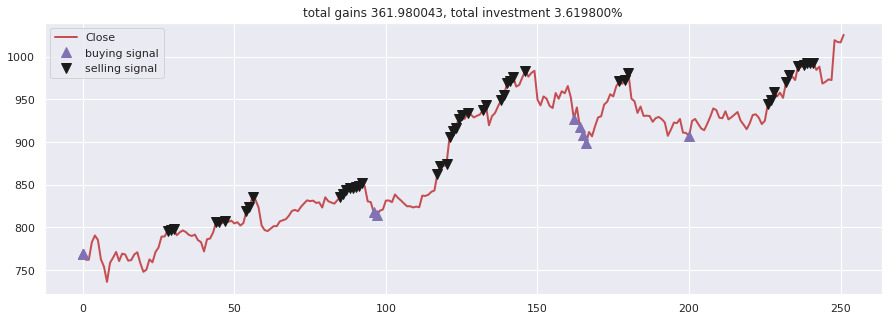1. Moving-average agent, moving-average-agent.ipynb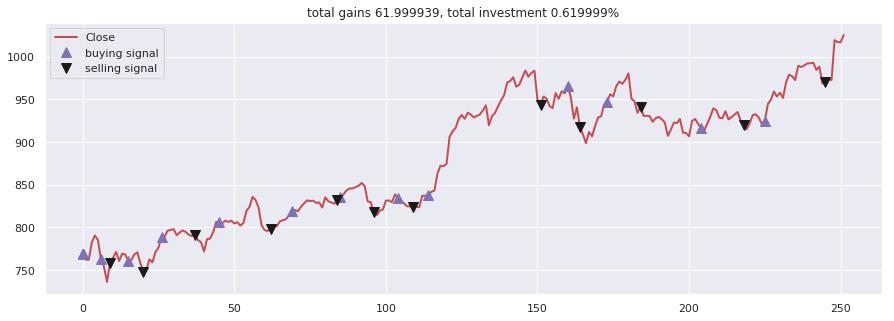1. Signal rolling agent, signal-rolling-agent.ipynb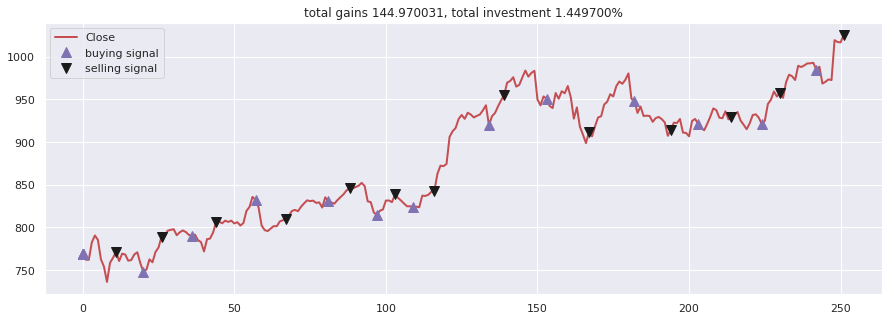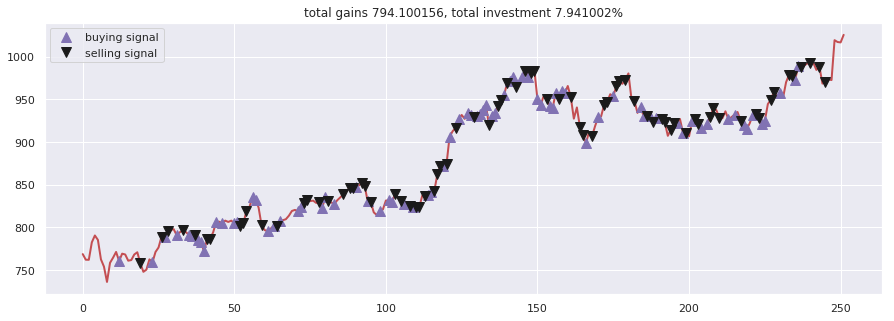1. Q-learning agent, q-learning-agent.ipynb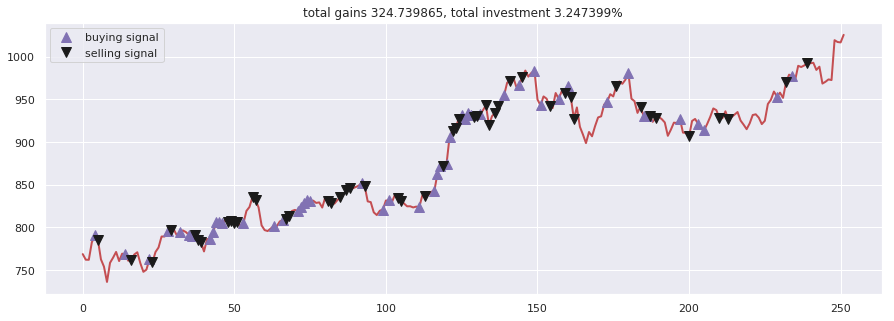1. Evolution-strategy agent, evolution-strategy-agent.ipynb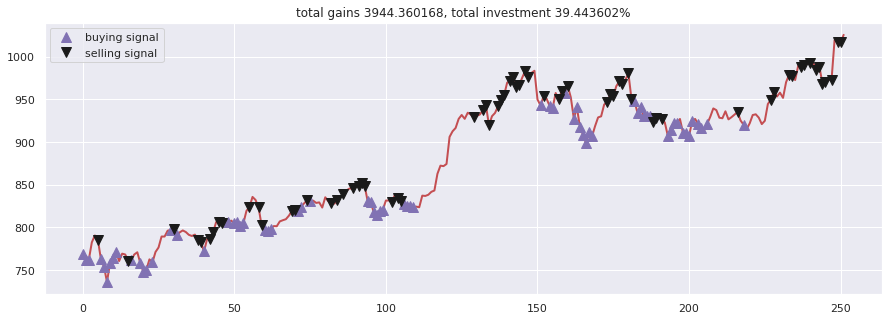1. Double Q-learning agent, double-q-learning-agent.ipynb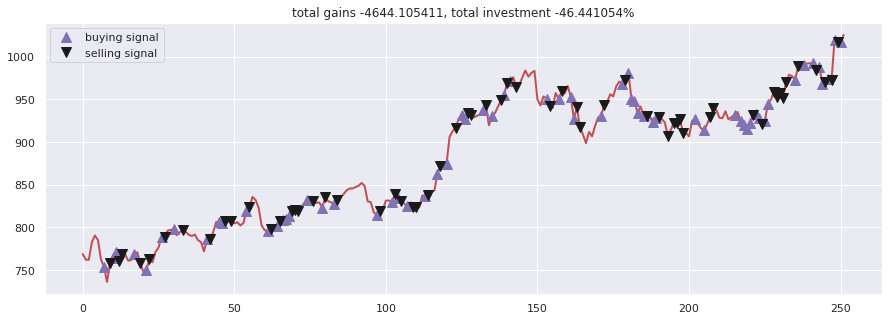1. Recurrent Q-learning agent, recurrent-q-learning-agent.ipynb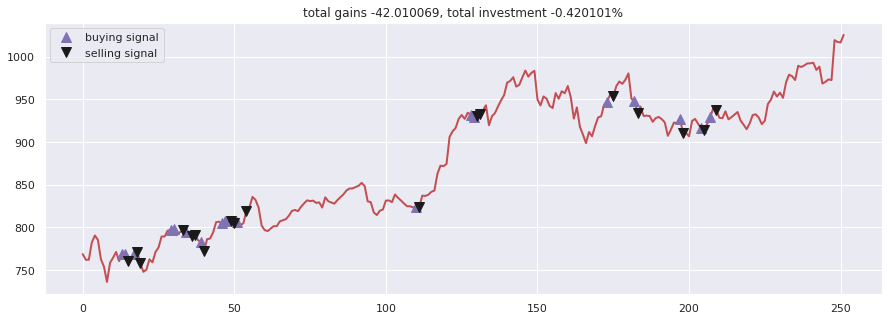1. Double Recurrent Q-learning agent, double-recurrent-q-learning-agent.ipynb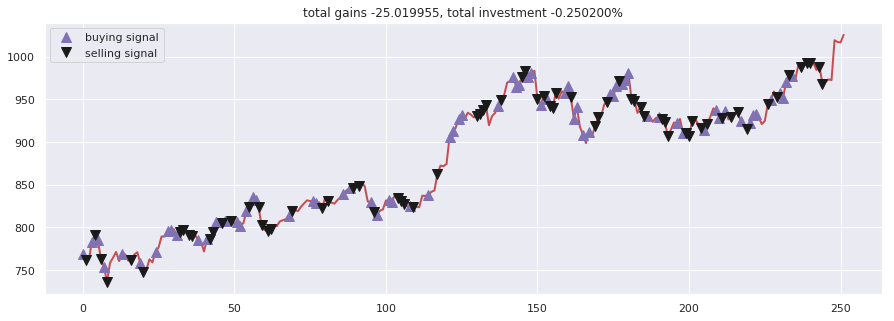1. Duel Q-learning agent, duel-q-learning-agent.ipynb1. Double Duel Q-learning agent, double-duel-q-learning-agent.ipynb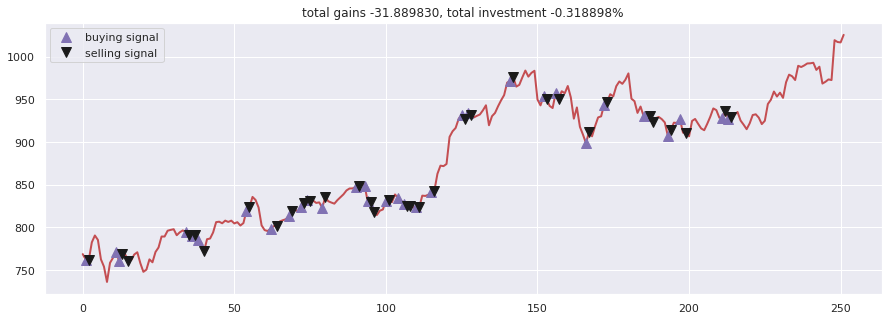1. Duel Recurrent Q-learning agent, duel-recurrent-q-learning-agent.ipynb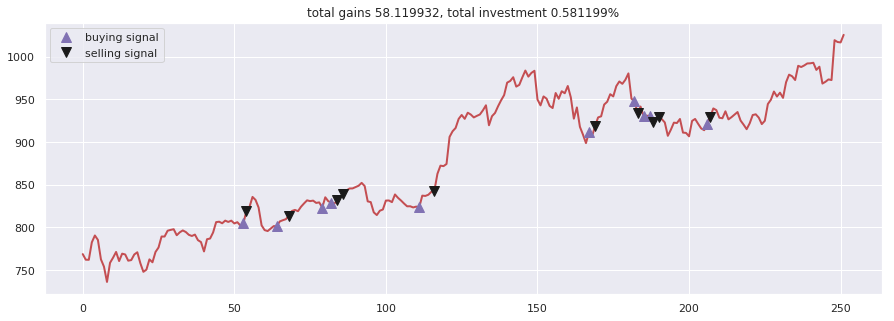1. Double Duel Recurrent Q-learning agent, double-duel-recurrent-q-learning-agent.ipynb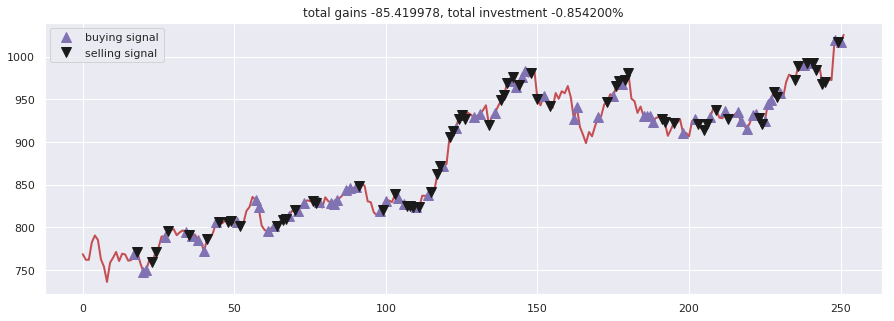1. Actor-critic agent, actor-critic-agent.ipynb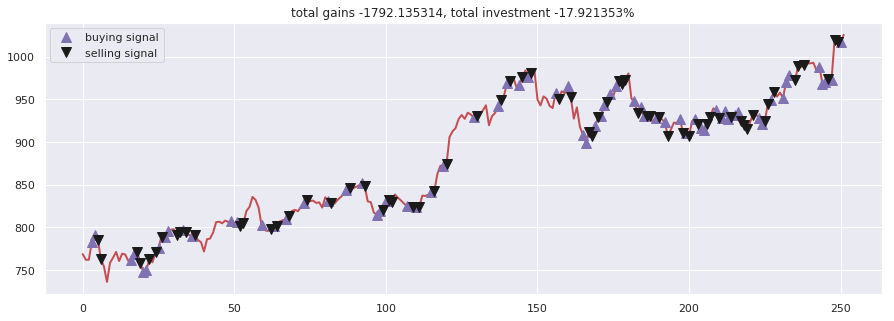1. Actor-critic Duel agent, actor-critic-duel-agent.ipynb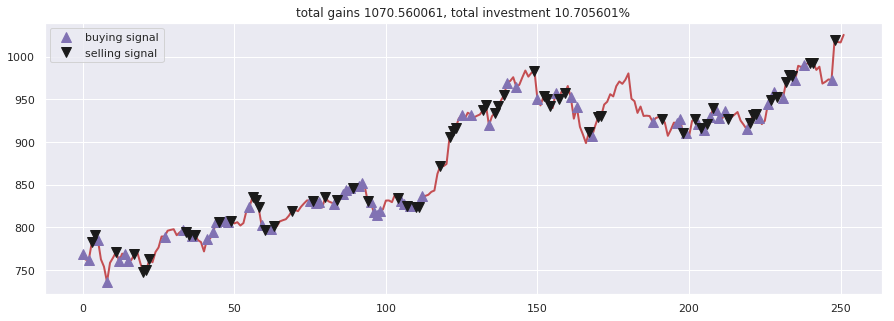1. Actor-critic Recurrent agent, actor-critic-recurrent-agent.ipynb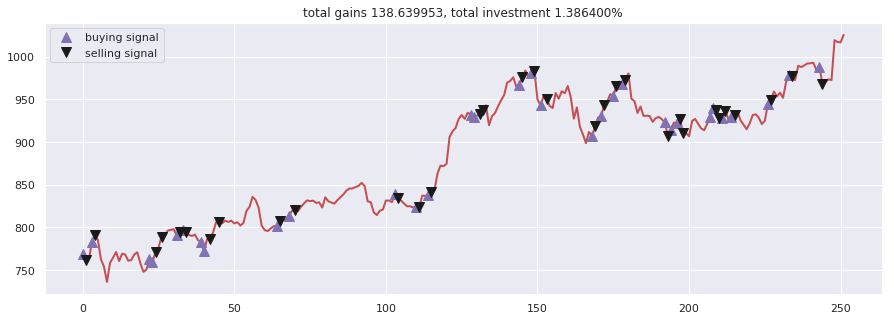1. Actor-critic Duel Recurrent agent, actor-critic-duel-recurrent-agent.ipynb1. Curiosity Q-learning agent, curiosity-q-learning-agent.ipynb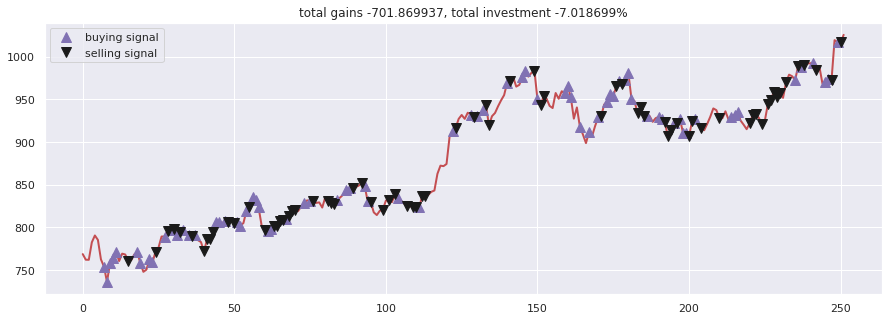1. Recurrent Curiosity Q-learning agent, recurrent-curiosity-q-learning.ipynb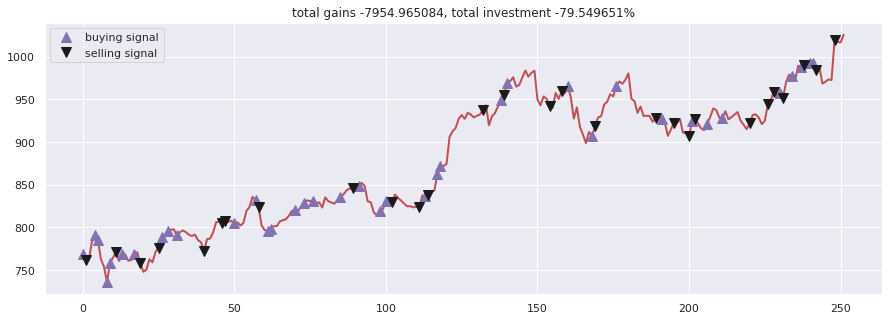1. Duel Curiosity Q-learning agent, duel-curiosity-q-learning-agent.ipynb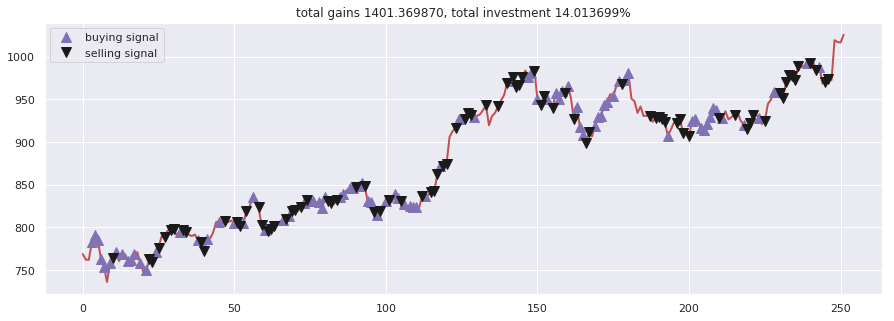1. Neuro-evolution agent, neuro-evolution.ipynb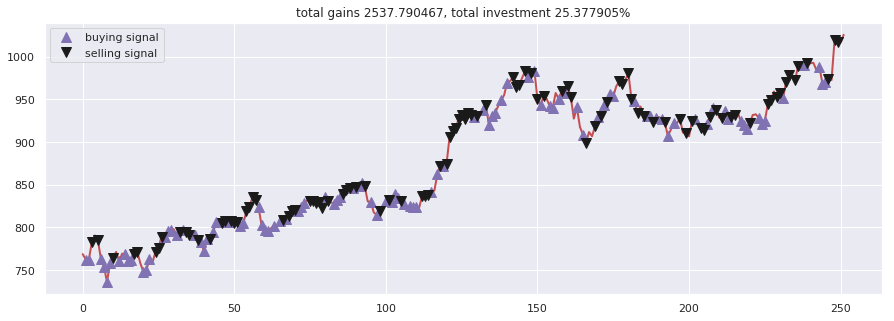1. Neuro-evolution with Novelty search agent, neuro-evolution-novelty-search.ipynb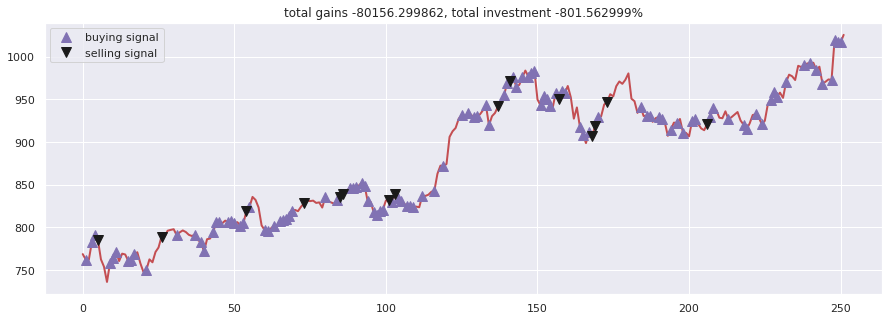1. ABCD strategy agent, abcd-strategy.ipynb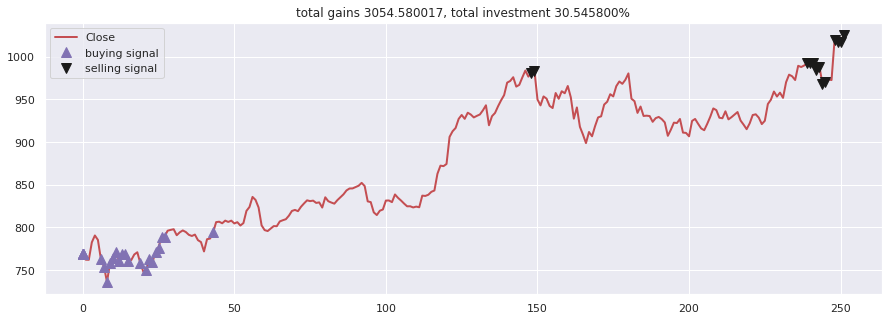Results free agent

This agent able to buy or sell N-units per transaction.

evolution strategy agent evolution-strategy-agent.ipynb

``````total gained 11037.529911, total investment 110.375299 %
``````

evolution strategy with bayesian agent evolution-strategy-bayesian-agent.ipynb

``````total gained 13295.469683, total investment 132.954697 %
``````Results signal prediction

LSTM Recurrent Neural NetworkLSTM Bidirectional Neural Network2-Path LSTM Recurrent Neural NetworkDeep Feed-forward Auto-Encoder Neural Network to reduce dimension + Deep Recurrent Neural Network + ARIMA + Extreme Boosting Gradient RegressorLSTM Sequence-to-Sequence Recurrent Neural NetworkLSTM Sequence-to-Sequence with Attention Recurrent Neural NetworkLSTM Sequence-to-Sequence with Attention Bidirectional Recurrent Neural NetworkEncoder-Decoder Feed-forward + LSTM Recurrent Neural NetworkAdaboost + Bagging + Extra Trees + Gradient Boosting + Random Forest + XGB